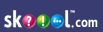Introduction Generix Content - Introduction
"Introduction" question number distribution across years
loading...
Financial Accounting index on skoool nigeriaWAEC questions for "Financial Accounting :: Introduction"
Q1

Which of the following is a basic accounting equation?

A

Assets = Capital + Liabilities

B

Capital = Assets + Liabilities

C

Liabilities = Assets + Capital

D

Assets = Liability - Equity

E

Answer: A

Introduction
Q2

Financial accounting information is for

A

internal use only

B

external use only

C

business use only

D

internal and external use

E

Answer: D

Introduction
Q3

The art of collecting, recording, presenting and interpreting accounting data is

A

cost accounting

B

management accounting

C

financial accounting

D

data processing

E

Answer: C

Introduction
Q4

The systemic recording of business transactions in monetary term is

A

auditing

B

book-keeping

C

debiting

D

crediting

E

Answer: B

Introduction
Q5

The art of recording transactions in books of account is known as

A

debiting

B

book-keeping

C

auditing

D

crediting

E

Answer: B

Introduction
Q6

The accounting equation is

A

Capital less Current Assets amounts to Liabilities

B

Assets less Liabilities amount to Capital

C

Fixed Assets and Current Liabilities amount to Capital

D

Capital and Assets amount to Liabilities

E

Answer: B

Introduction
Q7

The accounting equation is

A

assets and liabilities amount to capital

B

capital less assets amount to liabilities

C

assets less liabilities amount to capital

D

capital and assets amount to liabilities

E

fixed assets and current liabilities amount to capital

Answer: C

Introduction